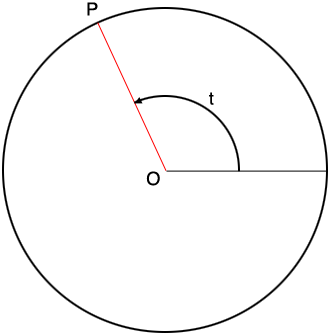SEARCH HOMEMath Central Quandaries & QueriesQuestion from anagha, a student: Hi, thank you for answering (beforehand) The other day I was just thinking of circles and I came across this peculiar thing. Most often they say that there are infinite number of points on the circumference. and, if it is true, if we spin the radius, the probability that it will point a a particular point is 1/infinity, right? It didn't make any sense to me and so, I thought there might be an explanation or something of the kind...?Hi Anagha,

I am going to draw the circle with its center at the origin $O$ in the Cartesian plane. I am going to identify a point $P$ on the circle by the measure $t$ of the angle between positive X-axis and the line segment from $P$ to $O,$ measured counterclockwise from the positive X-axis.The problem is that $P$ is an unknown point on the circumference of the circle and there is a spinner attached at $O.$ Spin the spinner. What is the probability that it stops, pointing at $P?$

Suppose the probability is $Pr.$ Start by dividing the circumference of the circle with the X-axis. The two segments of the circumference are specified by

$0^o \le t < 180^o \mbox{ and } 180^0 \le t < 360^{o}.$

These segments are the same size and hence the probability that the spinner points at the segment containing $P$ is $\large \frac{1}{2}.$ Thus $Pr \le \large \frac{1}{2}.$

Further subdivide the circumference by adding the Y-axis to obtain the four segments

$0^o \le t < 90^{o}, 90^o \le t < 180^{o}, 180^o \le t < 120^o\mbox{ and } 270^0 \le t < 360^{o}.$

These segments are the same size and hence the probability that the spinner points at the segment containing $P$ is $\large \frac{1}{4} = \large \frac{1}{2^2}.$ Thus $Pr \le \large \frac{1}{2^2}.$

Further subdivide the circumference by adding the lines through the origin at $45^o$ and $135^{o}.$ This subdivides the circumference into $2^3 = 8$ segments and hence $Pr \le \large \frac{1}{2^3}.$

You can continue this process indefinitely, each time doubling the number of segments from the previous step. Hence at the $n^{th}$ step we have that $Pr \le \large \frac{1}{2^n}.$ We also know that the probability of any event is between 0 and 1 and hence, for all positive integers $n,$

$0 \le Pr \le \frac{1}{2^n}.$

As $n$ approaches infinity the sequence

$\frac{1}{2}, \frac{1}{2^2}, \frac{1}{2^3}, \cdot \cdot \cdot, \frac{1}{2^n}, \cdot \cdot \cdot$

approaches zero and hence we are forced to conclude that $Pr = 0.$

I hope this helps,

HarleyMath Central is supported by the University of Regina and the Imperial Oil Foundation.